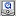- Computational Approach to Mathematical Sciences(\$B7W;;\$K\$h\$k?tM}2J3X\$NE83+(B), Video Archives -

\$B%W%m%8%'%/%H\$N%[!<%`(B(project home) (\$BD{@5Ey\$O\$3\$A\$i\$K7G<(\$5\$l\$k\$3\$H\$b\$"\$j\$^\$9(B(errata might be posted here))

 Lecture(\$B9V1i Title(\$B%?%\$%H%k(B): Parametrized Partition Relations Level: Research lecture\$B7A<0(B(format): QuickTime(\$BF|K\8l(B) , QuickTime(English) (Version 7 or later) Source: Abstract(\$BFbMF(B): We consider partitions relations defined on finite or infinite products of the Baire space. A set A of infinite sets of natural numbers is Ramsey if there is an infinite set X such that all of its infinite subsets are in A or none of them is in A, in this case we say that X is homogeneous for A. In Solovay's model all sets of reals (reals seen as infinite sets of integers) are Ramsey. Moreover, if for each x in the Cantor space we have a set of reals Ax, there is a perfect subset P of the Cantor space and an infinite set X of natural numbers such that X is homogeneous for all Ax with x in P. Stronger parametrized partition relations also hold in Solovay's model. Message from the speaker:

Keywords: serial=0048, type=research lecture
License(\$B%i%\$%;%s%9(B): No modification and free distribution. (creative commons, Attribution-NoDerivative works 2.1) Details

### Lecture video (\$B9V5A1GA|(B)

If you watch movies by streaming on Windows, please do not stop it by the "x" button. Your network connection might become very slow.
Windows\$B>e\$G(B Streaming \$B\$G1\Mw\$7\$F\$\$\$k>l9g(B, \$BDd;_\$9\$k\$H\$-\$O(B, \$BDd;_%\%?%s\$rI,\$:\$*\$7\$F\$/\$@\$5\$\$(B. x \$B\$GDd;_\$7\$J\$\$\$h\$&\$K(B. \$B%M%C%H%o!<%/\$N@\B3B.EY\$,6KC<\$KCY\$/\$J\$k>l9g\$,\$"\$j\$^\$9(B.)
To save movies, click the right button. (\$B1&%/%j%C%/\$G%@%&%s%m!<%IJ]B82DG=\$G\$9(B.)

```\$Id\$
```